In :
## Python II: Intermediate Tutorial
## University of Toronto Operations Research Group (UTORG) - Coding Month
## Teacher: Kyle E. C. Booth, Ph.D. Student - Industrial Engineering
## February 11, 2016

## Topics: Beginner tutorial referesher, data cleaning/alteration, JSON data structure, csv/text reading and writing,
## data science with pandas, numpy, matplotlib, machine learning classification with scikit.learn

## 1) Refresher - Data Types

number = 10
string = "ten"
list1 = [1,2,"three",4,5,6,7,"eight",9,10]
dictionary = {"one" : 1, "two": 2, "ten" : 10}
tuple1 = (1,2,3,"four",5,6,7,"eight",9,10)
print number, "\n", string, "\n", list1, "\n", dictionary, "\n", tuple1

10
ten
[1, 2, 'three', 4, 5, 6, 7, 'eight', 9, 10]
{'two': 2, 'ten': 10, 'one': 1}
(1, 2, 3, 'four', 5, 6, 7, 'eight', 9, 10)


In :
## 2) Refresher - Control Flows, if/elif/else

if number == 1:
print "The number is 1."
elif number == 10:
print "The number is 10."
else:
print "We don't know what the number is."

The number is 10.


In :
## 3) Refresher - Control Flows, 'for' loops

for i in range(number):
print i,
print "\n"

for item in list1:
print item,
print "\n"

for key in dictionary:
print dictionary[key], ## Unordered dictionary. Order according to hash table implementation.

0 1 2 3 4 5 6 7 8 9

1 2 three 4 5 6 7 eight 9 10

2 10 1


In :
## 4) Refresher - Control Flows 'while' loops

i = 0
while i < number:
print i,
i +=1
print "\n"

i = 0
while i < len(list1):
print list1[i],
i += 1

0 1 2 3 4 5 6 7 8 9

1 2 three 4 5 6 7 eight 9 10


In :
## 5) Refresher - Functions
## How would you describe this function?

def addTypes(x, y):
return x + int(y)

number = 10
string = "10"

print addTypes(number, string)

20


In :
## 6) List Comprehensions
## Create a list containing the numbers 1 through 10

## Typical Implementation:
list1 = []
for i in range(1,11):
list1.append(i)

print list1

## Using List Comprehensions:
list2 = [x for x in range(1,11)]

print list2

## Convenient way to reduce lines of code for clearer programs.

[1, 2, 3, 4, 5, 6, 7, 8, 9, 10]
[1, 2, 3, 4, 5, 6, 7, 8, 9, 10]


In :
## 7) List Operators

## Access the first item and the 6th item
print list2, list2

## Add an item to the end
list2.append(11)
print list2

## Remove an item from the end
list2.pop()
print list2

## Remove the first item and the 6th item
del list2, list2
print list2

## Replace value of an item (using comprehensions)
list2 = [str(item).replace('5','dog') for item in list2] ## Why do we cast str()?
print list2

## and many more... insert(), extend(), count(), etc.

1 6
[1, 2, 3, 4, 5, 6, 7, 8, 9, 10, 11]
[1, 2, 3, 4, 5, 6, 7, 8, 9, 10]
[2, 3, 4, 5, 6, 8, 9, 10]
['2', '3', '4', 'dog', '6', '8', '9', '10']


In []:
## 8) Opening Files - Python makes it easy.
## You have this file in your data folder

with open("data/test.txt") as f:
print(f.read())

## Please code this in your terminal and verify the output.

In []:
## 9) Opening Files - Store each line as a list element. (8 lines)

content = []
with open("data/test.txt") as f:
for line in f:
content.append(line)
print content

## Replace "serious" with "fun"? More list stuff!

newContent = [] ## Remember, everything is copies!
for item in content:
newContent.append(item.replace("serious","fun"))
print newContent

## Is there a better way to do this?

In []:
## 10) Let's do it a little better. (5 lines) TL;DR - Use list comprehensions

with open("data/test.txt") as f:
content = [line for line in f]
print content

## Replace "serious" with "fun"? More list stuff!

newContent = [item.replace("serious", "fun") for item in content]
print newContent

In :
## 11) CODING ACTIVITY: DATA CLEANING/ALTERATION

## Time: 15 Minutes

## Read in the 'nameSwap.txt' file to a Python list (line by line). Replace all instances of "William Bradley" with ''
## "Brad" with your first name, "Pitt" with your last name, and "American" with Canadian.
## Bonus: You also need to number the lines in the ouput - there should be 5 lines. (hint: list.insert(index,value))
## Don't number lines without content! (there should be two empty lines)
## Store this text in a new list, and then output this information to a file (in the same directory)
## called 'nameSwapped.txt'
##
## Hint:
## with open(file, 'w') as f:
##    f.write(  )
## to write to a file.

In :
## Solution (10 lines)

with open("data/nameSwap.txt") as f:
content = [line.strip() for line in f]

newContent = [item.replace("William Bradley",'').replace("Brad", "Kyle").replace("Pitt", "Booth").replace("American", "Canadian") \
for item in content]

index = 1
with open("data/nameSwapped.txt", 'w') as f:
for item in newContent:
if item:
f.write("%d. %s\n\n" % (index, item))
index += 1

In :
## 12) Data Structures: Dictionaries
## These are pretty neat, too.

actualDictionary = {"car":"an automobile", "cat":"meow", "engineer":"often stays up all night"}

## Then we can query it based on KEYS
## Note that dictionaries are mutable, and anything can go in them!

print actualDictionary

print actualDictionary["engineer"]

## Loop through it with for control

for key in actualDictionary:
print actualDictionary[key]

## Add a value (we could alter as well)
actualDictionary["UofT Ranking"] = 1

## Print all key/value pairs (this can be convenient)
for key, value in actualDictionary.iteritems():
print key + ":", value

{'car': 'an automobile', 'engineer': 'often stays up all night', 'cat': 'meow'}
often stays up all night
an automobile
often stays up all night
meow
car: an automobile
engineer: often stays up all night
UofT Ranking: 1
cat: meow


In :
## 13) We can read a JSON data file directly to a dictionary or list
## JSON is a very popular data interchange format, similar to XML or Google Protocol Buffers
## Documentation: http://www.json.org/

import json
import pprint

with open("data/test.json") as json_file:
json_data = json.load(json_file)
pprint.pprint(json_data)

print "\n", json_data["created"]["changeset"]

{u'created': {u'changeset': u'8139974',
u'timestamp': u'2011-05-14T11:45:29Z',
u'uid': u'260682',
u'user': u'monxton',
u'version': u'5'},
u'id': u'132707',
u'pos': [50.9454657, -1.4775675],
u'type': u'node'}

8139974


In []:
## 14) CODING ACTIVITY: NAVIGATING DICTIONARIES & JSON

## Time: 10 Minutes

## Read random.json file in using import json.
## Using your knowledge of dictionaries, write code that will
## output a list containing Vance Rogers eye colour, email and a list of his friends (list within a list).

In :
## Solution (11 lines) What could we use to improve this?

import json
import pprint

with open("data/random.json") as json_file:
json_data = json.load(json_file)

solution = []
for item in json_data:
if item["name"] == "Vance Rogers":
solution.append(item["eyeColor"])
solution.append(item["email"])
friends = []
for friend in item["friends"]:
friends.append(friend["name"])
solution.append(friends)

print "Solution List: ", solution

Solution List:  [u'brown', u'vancerogers@comtest.com', [u'Diaz Burke', u'Helen Greer', u'Nunez Copeland']]


In :
## 15) Numpy & Matplotlib
## Let's get plotting!
## Documentation: http://matplotlib.org/

%matplotlib inline
import numpy as np
import matplotlib.pyplot as plt

x = [1,2,3,4,5,6,7,8,9,10]
y = [z**2 for z in x]

plt.scatter(x, y)
plt.show()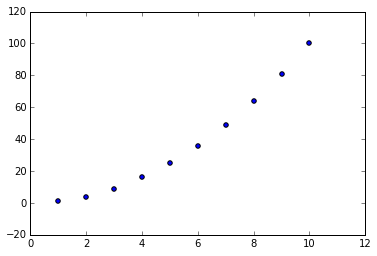In :
## 16) Another plot
## This one is grabbed from matplotlib.org

N = 50 ## number of data points
x = np.random.rand(N) ## Randomly generated between in [0,1]
y = np.random.rand(N)
colors = np.random.rand(N)
area = np.pi * (15 * np.random.rand(N))**2  # 0 to 15 point radiuses

plt.scatter(x, y, s=area, c=colors, alpha=0.5)
plt.show()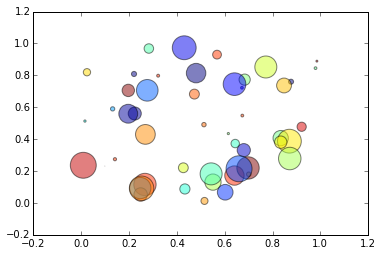In :
## 17) Let's bring these two plots together as subplots to a larger plot.

## First Plot
x = [1,2,3,4,5,6,7,8,9,10]
y = [z**2 for z in x]
plt.subplot(2, 1, 1) # rows, columns, position
plt.scatter(x, y)

## Second Plot
N = 50
x = np.random.rand(N) # Here we call Numpy with "np"
y = np.random.rand(N)
colors = np.random.rand(N)
area = np.pi * (15 * np.random.rand(N))**2  # 0 to 15 point radiuses
plt.subplot(2, 1, 2)
plt.scatter(x, y, s=area, c=colors, alpha=0.5)

plt.show()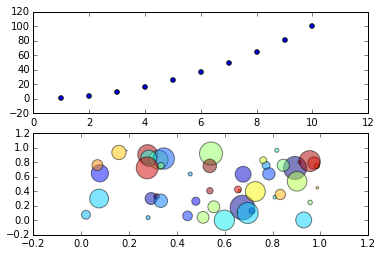In :
## 18) CODING ACTIVITY: PLOT THE FIBONACCI SEQUENCE

## Time: 10 Minutes

## For the x-axis, use [1,2,3,4,5,6,7,8,9,10,...,25]
## for the y-axis, use the Fibonacci Sequence defined as the sum of the previous two numbers (e.g. 1, 1, 2, 3 etc.)
## Create two subplots: 1x normal, 1x in logscale (in the y-axis)
## plt.yscale('log')

In :
## Solution:

fibonacci = []
x = []
for i in range(25):
x.append(i+1)
if i > 1:
fibonacci.append(fibonacci[i-1]+fibonacci[i-2])
else:
fibonacci.append(1)

plt.subplot(2, 1, 1)
plt.scatter(x, fibonacci)

plt.subplot(2, 1, 2)
plt.yscale('log')
plt.scatter(x, fibonacci)

plt.show()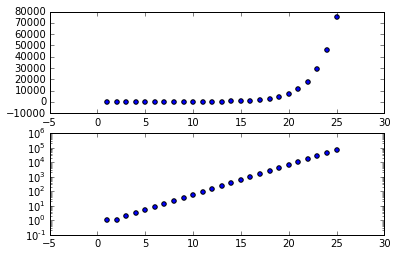In :
## 19) CSV Read with Pandas
## Welcome to Dataframes (df)

## Use Pandas to quickly read data into dataframe
## Pandas documentation: http://pandas.pydata.org/

import pandas as pd

df = pd.read_csv('data/test.csv', index_col=False, header=0);

print df

print df.head(1)

   ID  age  height  weight
0   1   27      80     170
1   2   26      76     165
2   3   34      54     130
ID  age  height  weight
0   1   27      80     170


In :
## 21) CODING ACTIVITY: RED WINE EXPLORATORY ANALYSIS

## Time: 10 Minutes

## Read the 'wine.csv' file into a Pandas dataframe
## Plot (scatter) the relationship between alcohol content (x-axis) and wine quality (y-axis)
## What do you see?
## Bonus: Include a trendline!

In :
## 20) Plotting with Pandas

## Use Matplotlib to create scatterplot
plt.scatter(df['ID'], df['age'])

## We can add a linear trendline using Numpy (as np)
z = np.polyfit(df['ID'], df['age'], 1)
p = np.poly1d(z)

plt.plot(df['ID'],p(df['ID']))
plt.show()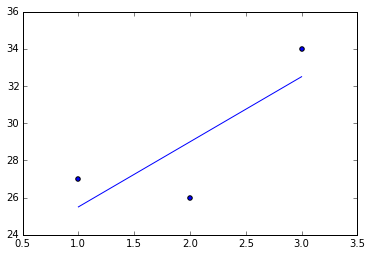In :
## Solution

df = pd.read_csv('data/wine.csv', index_col=False, header=0);

plt.scatter(df['quality'], df['alcohol'])
z = np.polyfit(df['quality'], df['alcohol'], 1)
p = np.poly1d(z)
plt.plot(df['quality'],p(df['quality']))

plt.title('Exploring Red Wine')
plt.xlabel('quality')
plt.ylabel('alcohol %')

plt.show()

## Is this very clear? What would be nicer?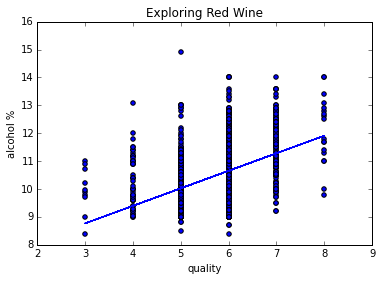In :
## Better Solution (perhaps)

## Let's group the alcohol values as averages for each quality value
df = df.groupby('quality', as_index=False)['alcohol'].mean()

## Plot the new dataframe
plt.scatter(df['quality'], df['alcohol'])
z = np.polyfit(df['quality'], df['alcohol'], 1)
p = np.poly1d(z)
plt.plot(df['quality'],p(df['quality']))

plt.title('Exploring Red Wine')
plt.xlabel('quality')
plt.ylabel('alcohol %')

plt.show()

## Much clearer!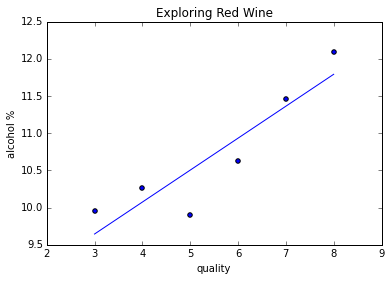In :
## 22) Final Chapter (Brief) Machine Learning with Scikit-Learn
## Documenation: http://scikit-learn.org/

from sklearn import datasets
from sklearn.naive_bayes import GaussianNB
from sklearn import tree
from sklearn.ensemble import RandomForestClassifier

iris = datasets.load_iris() ## Famous dataset: en.wikipedia.org/wiki/Iris_flower_data_set

#clf = GaussianNB()
#clf = tree.DecisionTreeClassifier()
clf = RandomForestClassifier(n_estimators=10)

## Fit the classification method to the data
clf.fit(iris.data, iris.target)

## Ask the classification method to predict 'target' based on 'data'
y_pred = clf.predict(iris.data)

print "Target Data: \n"
print iris.target
print "\nClassifier Predictions: \n"
print y_pred

print("\nNumber of mislabeled points out of a total %d points : %d" % (iris.data.shape,(iris.target != y_pred).sum()))

## What is an issue with this?

Target Data:

[0 0 0 0 0 0 0 0 0 0 0 0 0 0 0 0 0 0 0 0 0 0 0 0 0 0 0 0 0 0 0 0 0 0 0 0 0
0 0 0 0 0 0 0 0 0 0 0 0 0 1 1 1 1 1 1 1 1 1 1 1 1 1 1 1 1 1 1 1 1 1 1 1 1
1 1 1 1 1 1 1 1 1 1 1 1 1 1 1 1 1 1 1 1 1 1 1 1 1 1 2 2 2 2 2 2 2 2 2 2 2
2 2 2 2 2 2 2 2 2 2 2 2 2 2 2 2 2 2 2 2 2 2 2 2 2 2 2 2 2 2 2 2 2 2 2 2 2
2 2]

Classifier Predictions:

[0 0 0 0 0 0 0 0 0 0 0 0 0 0 0 0 0 0 0 0 0 0 0 0 0 0 0 0 0 0 0 0 0 0 0 0 0
0 0 0 0 0 0 0 0 0 0 0 0 0 1 1 1 1 1 1 1 1 1 1 1 1 1 1 1 1 1 1 1 1 1 1 1 1
1 1 1 1 1 1 1 1 1 1 1 1 1 1 1 1 1 1 1 1 1 1 1 1 1 1 2 2 2 2 2 2 2 2 2 2 2
2 2 2 2 2 2 2 2 2 2 2 2 2 2 2 2 2 2 2 2 2 2 2 2 2 2 2 2 2 2 2 2 2 2 2 2 2
2 2]

Number of mislabeled points out of a total 150 points : 0


In :
## 23) Red Wine - Can we guess a wine's quality?

df = pd.read_csv('data/wine.csv');

## Remove the ID column
df = df.drop('ID', axis=1)

## Print only first 5 results
print df.head(5)

   fixed.acidity  volatile.acidity  citric.acid  residual.sugar  chlorides  \
0            7.4              0.70         0.00             1.9      0.076
1            7.8              0.88         0.00             2.6      0.098
2            7.8              0.76         0.04             2.3      0.092
3           11.2              0.28         0.56             1.9      0.075
4            7.4              0.70         0.00             1.9      0.076

free.sulfur.dioxide  total.sulfur.dioxide  density    pH  sulphates  \
0                   11                    34   0.9978  3.51       0.56
1                   25                    67   0.9968  3.20       0.68
2                   15                    54   0.9970  3.26       0.65
3                   17                    60   0.9980  3.16       0.58
4                   11                    34   0.9978  3.51       0.56

alcohol  quality
0      9.4        5
1      9.8        5
2      9.8        5
3      9.8        6
4      9.4        5


In :
## Train your model
from sklearn import datasets
from sklearn.naive_bayes import GaussianNB
from sklearn import tree
from sklearn.ensemble import RandomForestClassifier

data = df.values

clf = GaussianNB()
#clf = tree.DecisionTreeClassifier()
#clf = RandomForestClassifier(n_estimators=10)

clf = clf.fit(data[0:1200,0:10], data[0:1200,11])

In :
## Output the predictions

output = clf.predict(data[1200:1598,0:10])

from sklearn.metrics import accuracy_score

print output
print accuracy_score(data[1200:1598, 11], output)

[ 6.  6.  6.  6.  6.  6.  6.  5.  6.  7.  5.  6.  5.  6.  6.  7.  6.  6.
6.  7.  6.  6.  6.  7.  6.  5.  5.  5.  7.  6.  6.  6.  6.  6.  6.  6.
6.  6.  5.  7.  5.  6.  6.  5.  6.  5.  6.  5.  6.  6.  6.  5.  6.  6.
7.  6.  5.  7.  5.  5.  6.  5.  5.  6.  6.  6.  6.  7.  6.  7.  6.  6.
6.  6.  6.  5.  7.  6.  5.  5.  6.  6.  6.  5.  6.  5.  6.  7.  5.  5.
6.  5.  7.  5.  5.  5.  5.  7.  4.  4.  5.  5.  7.  8.  5.  6.  5.  7.
5.  5.  6.  6.  4.  5.  5.  5.  5.  5.  5.  6.  5.  5.  7.  7.  6.  6.
6.  6.  6.  5.  5.  6.  6.  5.  5.  6.  6.  6.  6.  6.  6.  6.  6.  6.
5.  5.  6.  6.  6.  7.  6.  6.  6.  6.  5.  6.  6.  6.  6.  5.  5.  5.
5.  5.  6.  6.  5.  5.  5.  7.  4.  6.  4.  5.  5.  6.  6.  6.  5.  6.
6.  6.  5.  5.  6.  6.  6.  6.  7.  6.  6.  6.  6.  5.  4.  6.  6.  6.
5.  6.  5.  5.  7.  6.  6.  7.  6.  8.  6.  8.  5.  6.  6.  5.  7.  7.
7.  6.  6.  6.  6.  5.  5.  6.  5.  5.  7.  6.  7.  6.  6.  5.  7.  5.
6.  6.  5.  4.  4.  5.  6.  5.  5.  6.  5.  5.  5.  5.  6.  5.  6.  7.
5.  6.  5.  7.  7.  6.  5.  7.  6.  6.  6.  6.  5.  5.  5.  5.  5.  6.
6.  7.  6.  6.  6.  7.  6.  7.  4.  6.  7.  6.  4.  7.  7.  5.  5.  5.
6.  7.  6.  6.  6.  5.  6.  6.  5.  6.  4.  6.  6.  6.  6.  7.  5.  5.
5.  5.  5.  7.  7.  5.  5.  7.  7.  7.  6.  6.  5.  6.  6.  5.  6.  6.
5.  4.  4.  6.  6.  6.  4.  7.  6.  6.  6.  6.  7.  7.  7.  7.  7.  6.
6.  5.  6.  6.  5.  6.  6.  6.  5.  5.  6.  5.  4.  7.  5.  4.  5.  5.
5.  5.  5.  5.  5.  6.  5.  5.  5.  6.  7.  7.  5.  7.  4.  6.  5.  7.
6.  6.  6.  6.  6.  5.  7.  6.  6.  6.  6.  6.  5.  5.  5.  6.  4.  8.
5.  5.]
0.417085427136


In :
## 23) How about survivors for 'The Titanic'?
## Give this a try on your own!

df = pd.read_csv('data/titanic.csv');

print df.head(5)

## What do we notice?

   PassengerId  Survived  Pclass  \
0            1         0       3
1            2         1       1
2            3         1       3
3            4         1       1
4            5         0       3

Name     Sex  Age  SibSp  \
0                            Braund, Mr. Owen Harris    male   22      1
1  Cumings, Mrs. John Bradley (Florence Briggs Th...  female   38      1
2                             Heikkinen, Miss. Laina  female   26      0
3       Futrelle, Mrs. Jacques Heath (Lily May Peel)  female   35      1
4                           Allen, Mr. William Henry    male   35      0

Parch            Ticket     Fare Cabin Embarked
0      0         A/5 21171   7.2500   NaN        S
1      0          PC 17599  71.2833   C85        C
2      0  STON/O2. 3101282   7.9250   NaN        S
3      0            113803  53.1000  C123        S
4      0            373450   8.0500   NaN        S


In :
## 21) CODING ACTIVITY: MACHINE LEARNING: TITANIC SURVIVORS

## Time: 10 Minutes

## Use the 'titanic.csv' file
## Figure out what columns are useful, what aren't
## Experiment with different classifiers, paramters, GridSearch, etc.

In :
## Continued - What should we remove?

df = df.drop(['Name', 'Ticket', 'Cabin'], axis=1)
df = df.dropna() ## Drop all NaN Values

print df.head(5)

## Anything else? Etc...

   PassengerId  Survived  Pclass     Sex  Age  SibSp  Parch     Fare Embarked
0            1         0       3    male   22      1      0   7.2500        S
1            2         1       1  female   38      1      0  71.2833        C
2            3         1       3  female   26      0      0   7.9250        S
3            4         1       1  female   35      1      0  53.1000        S
4            5         0       3    male   35      0      0   8.0500        S


In []:
## Thanks for attending! UTORG will announce future sessions, so please stay tuned.